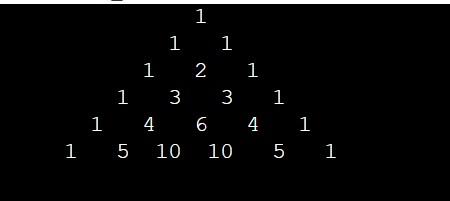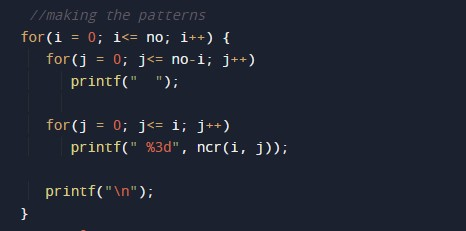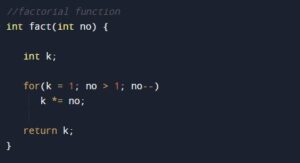C Program to Print Pascal Triangle

In this tutorial you will learn about the C Program to Print Pascal Triangle and its application with practical example.

C Program to Print Pascal Triangle

In this tutorial, we will learn to create a C program that will create Pascal Triangle using C programming.

Prerequisites

Before starting with this tutorial we assume that you are best aware of the following C programming topics:

• Operators in C Programming.
• Basic Input and Output function in C Programming.
• Basic C programming.
• Basic arithmetic operations.
• For loop in C Programming.

Create a Pascal Triangle:-

The C language is a very powerful programming language. In C programming we can perform many operations with the help of codings. The c language is very easy to create any pattern. In this program, we will learn to create pascal’s triangle with the help of some code.

The pascal’s triangle contains binomial coefficients.

Program:-

To Create a Pascal Triangle.

Output:-In the above program, we have first initialized the required variable.• i = it will hold the integer value to control parent for loop.
• j = it will hold the integer value to control child for loop.
• k = it will hold the integer value to control child for loop.

Initializing the first parent for loop.For Loop for factorial.In this tutorial we have learn about the C Program to Print Pascal Triangle and its application with practical example. I hope you will like this tutorial.